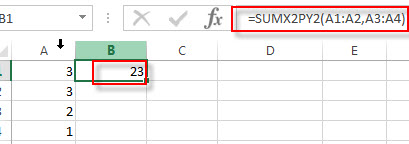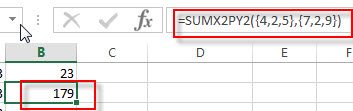## Excel SUMXMY2 Function

This post will guide you how to use Excel SUMXMY2 function with syntax and examples in Microsoft excel.

### Description

The Excel SUMXMY2 function returns the sum of the squares of differences between corresponding values in two given arrays and then return the sum of the result. You can use the SUMXMY2 function to calculate the sum of the squares of the differences between two supplied arrays in excel.

The SUMXMY2 function is a build-in function in Microsoft Excel and it is categorized as a Math and Trigonometry Function.

The SUMXMY2 function is available in Excel 2016, Excel 2013, Excel 2010, Excel 2007, Excel 2011 for Mac.

### Syntax

The syntax of the SUMXMY2 function is as below:

`=SUMXMY2 (array_x, array_y)`

Where the SUMXMY2 function arguments are:

• array_x -This is a required argument. The first array or range of values.
• array_y -This is a required argument. The second array or range of values

Note:

• The arguments can be numbers or names, arrays or references that contain numbers.
• empty cells, logical values , text or error values in the array or reference will be ignored.
• if array_x and array_y arguments have different lengths, the Function will return #N/A Error.
• The equation for the sum of the difference of squares is:

### Excel SUMXMY2 Function Examples

The below examples will show you how to use Excel SUMXMY2 Function to calculate the sum of the squares of the differences between two given array values in Excel.

1# to sum of squares of differences of the two arrays A1:A2 and A3:A4 , enter the following formula in Cell B1.

`=SUMXMY2(A1:A2,A3:A4)`2# to sum of squares of differences of the two arrays constants {4,2,5} and {7,2,9}, enter the following formula in Cell B2.

`=SUMXMY2({4,2,5},{7,2,9})`### Related Functions

• Excel SUM Function
The Excel SUM function will adds all numbers in a range of cells and returns the sum of these values. You can add individual values, cell references or ranges in excel.The syntax of the SUM function is as below:= SUM(number1,[number2],…)…
• Excel SUMIF Function
The Excel SUMIF function sum the numbers in the range of cells that meet a single criteria that you specify. The syntax of the SUMIF function is as below:=SUMIF (range, criteria, [sum_range])…
• Excel SUMIFS Function
The Excel SUMIFS function sum the numbers in the range of cells that meet a single or multiple criteria that you specify. The syntax of the SUMIFS function is as below:=SUMIFS (sum_range, criteria_range1, criteria1, [criteria_range2, criteria2], …)…
• Excel SUMSQ Function
The Excel SUMSQ function returns the sum of squares of a given set of arguments.The syntax of the SUMSQ function is as below:=SUMSQ(number1, [number2], …)
• Excel SUMX2MY2 Function
The Excel SUMX2MY2 function returns the sum of the difference of squares between two supplied arrays.The syntax of the SUMX2MY2 function is as below:=SUMX2MY2 (array_x, array_y)…
• Excel SUMX2PY2 Function
TThe Excel SUMX2PY2 function returns the sum of the sum of squares of corresponding items in two supplied arrays. The syntax of the SUMX2PY2 function is as below:=SUMX2PY2 (array_x, array_y)…